or# Inverse Trigonometric Functions NCERT Solutions : Class 12 Maths

## NCERT Solutions for Class 12 Maths : Inverse Trigonometric FunctionsFilter
Filters :
Classes
•
•
•
•
•
•
•
Chapters
•
•
•
•
•
•
•
•
•
•
• 3 More
Exercises
•
•
•
•

Doubtnut one of the best online education platform provides free NCERT Solutions of Maths for Class 12 Inverse Trigonometric Functions which are solved by our maths experts as per the NCERT (CBSE) guidelines. We provide the solutions as video solutions in which concepts are also explained along with answer which will help students while learning , preparing for exams and in doing homework. These Solutions will help to revise complete syllabus and score more marks in examinations.

Get here free, accurate and comprehensive NCERT Solutions for Class 12 Maths Inverse Trigonometric Functions which have been reviewed by our maths counsellors as per the latest edition following up the CBSE guidelines. We provide video solutions in which solutions to all the questions of NCERT Class 12 Maths textbook are explained in step by step and detailed way.

## The Topics of the Chapter Inverse Trigonometric Functions are :

RELATING DIFFERENT INVERSE TRIGONOMETRIC FUNCTIONS

PROPERTIES OF INVERSE TRIGONOMETRIC FUNCTIONS

PRINCIPAL VALUE OF THE FUNCTION

INVERSE TRIGONOMETIRC FUNCTIONS

TRIGONOMETIRC FUNCTIONS

It contains these exercises along with solved examples. All the exercises are solved in the video. Select the exercise to view the solutions exercisewise of the Chapter Inverse Trigonometric Functions:

## NCERT Solutions Class 12 Maths Chapter Inverse Trigonometric Functions Exercises:

We have covered all the exercises and also Solved examples in the videos. Along with the practise exercise Students should also practise solved examples to clear the concepts of the Inverse Trigonometric Functions. If incase you have any doubt you can watch the solutions for the given questions.Watch the solutions for the given questions in which it is also explained in the video steps to solve the questions along with answers.

# NCERT Class 12 Solution Chapter 2 Inverse Trigonometric Functions

Until now, students must be familiar with different trigonometric functions, the composition of functions and their application in different questions. The students should be knowing the different relation between length, breadth, and hypotenuse of a right-angle triangle. So now, here we will study about a different part, which is related to trigonometric functions, that is ‘inverse trigonometric functions’ and everything related to it as a whole in the form of topics and subtopics for the classified and easy understanding of the topic. The NCERT textbook gives the best and the perfect amount of information that is authentic for each and every topic related to the chapter, and for that sole reason, students should go for NCERT content always for the reference on any topic of any subject. Students should be thorough with each mathematical concept mentioned in the NCERT book before appearing for any examination and also should revise at least twice before appearing for the examination.

Though there are a number of books of different authors and publications circulated around in the market, NCERT is one such which is most preferred by the students as it provides a thorough and detailed explanation of each and every topic related to the subject. NCERT is the core study material as it is prescribed the CBSE board. As it has the motive to provide knowledge to maximum student possible and not rob them in any way. It is highly cheap and filled with colors to attract the students and make them interested in the topic unlike other books, which are all monotonous in appearances, which makes it boring to read. This book is really good to enhance the skills of the students in mathematics and help students of class 12 to do really good in their academics.

Doubtnut, an online platform, provides class 12 maths solution for NCERT textbook. The solutions are provided in video format and pdf format.  The video solutions and pdf solutions enable the students to understand the topic, chapter, and the overall subject comprehensively. We hereby also provide video lectures for every chapter and make sure that every topic is covered and made clear by experts in a fun and easy way possible. The topics and questions related to it are solved in the lectures and important questions are discussed as well according to the examination pattern. Students must download Doubtnut mobile app or reach us through our website for any explanation needed regarding any topic or any chapter and make their learning more interesting and beneficial.

## Topics & Subtopics of NCERT Class 12 Maths Chapter 2 – Inverse Trigonometric Functions

### INVERSE FUNCTIONS

#### Definition

If a function, say f is one to one and onto from A to B, then function g associates each element yÏµB to one and only one element xÏµA, such that y=f(x), then g is called the inverse function of f,

Denoted by x= g(y)

Usually, we denote g = 1/f

Therefore, x = 1/f(y)

As we know trigonometric functions are periodic functions and hence we can conclude that not all trigonometric functions are bijections (one-one onto function) thus their inverses do not exist. However, if we restrict their domains and co- domains, they can be made bijections and so we can obtain their inverses.

### Domain and Range of Inverse Trigonometric Functions

1. If sin y = x, then = 1/sin x under certain conditions.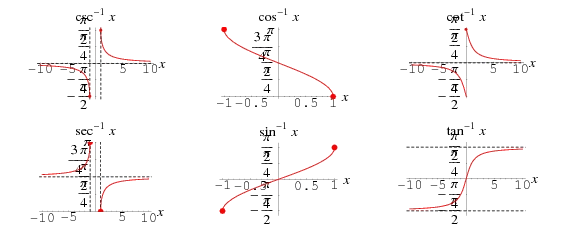←principal value branch of y=1/sin x

Because, -1 sin y ≤ 1; but sin y= x

Therefore, -1 ≤ x ≤ 1

Also, sin y = -1 = y = -π/2

And sin y = 1 = y = π/2

These restrictions on the values of x and y make the function sin y = x, one-one and onto so that the inverse function exists, that is y = 1/sin x makes sense

Domain: x Ïµ [-1, 1]

Range:  y Ïµ [-π/2, π/2]

2. Let cos y = x then y = 1/cos x under certain conditions,

Because -1 ≤ cos y ≤ 1

Therefore -1 ≤ x ≤ 1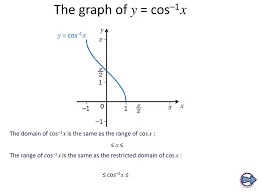Also, cos y = -1 = y = π

And cos y = 1 = y = 0

0≤y≤π

These restrictions on the values of x and y make the function of cos y = x one -one and onto so that the inverse function exists, therefore, y= 1/cos x that makes sense

Thus, Domain: x Ïµ [-1, 1]

Range:   y Ïµ [0, π]

3. If tan y = x then y = 1/tan x, under certain conditions.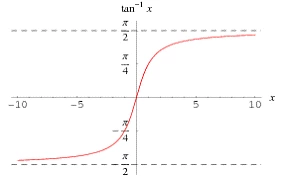Because tan y Ïµ R

x Ïµ R

Also, -∞ < tan y < ∞ = -π/2 < y < π/2

These restrictions on x and y makes the function,

Tan y = x one-one and onto so that the inverse function exists, that is

y= 1/tan x is meaningful.

Thus, domain: x Ïµ R, range: y Ïµ (-π/2, π/2)

4. If cot y = x, then y = 1/cot x, under certain conditions.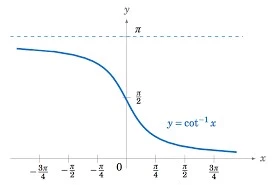Because, cot y Ïµ R;

Therefore, x Ïµ R;

Also, -∞ < cot y < ∞ = 0

These restrictions on x and y make the function,

Cot y = x one – one and onto so that the inverse function exists therefore;

y = 1/cot x is meaningful.

Domain: x Ïµ R

Range: y Ïµ (0, π)

5. If sec y = y, then y = 1/ sec x, where |x| ≥ 1 and 0≤ y ≤ π’

y ≠ π/2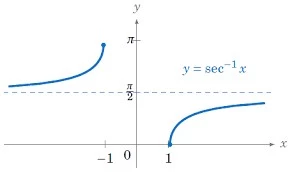Here, Domain: x Ïµ R -(-1, 1)

Range: y Ïµ [0, π] – {π/2}

6. If cosec y = x then y = 1/cosec x,

Where |x| ≥ 1 and -π / 2 ≤ y ≤ π/2, y≠0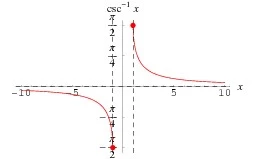Here, Domain: x Ïµ R -(-1,1)

Range: y Ïµ [-π/2, π/2] – {0}

Principal Values and Domain of Inverse Trigonometric / Circular Functions: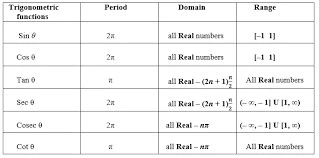NOTE:

1. If no branch of an inverse trigonometric function is mentioned, then it means the principal value branch of the function.

2. No inverse function is periodic.

3. 1st quadrant is common to the range of all inverse trigonometric functions.

4. 3rd quadrant is not used in an inverse trigonometric function.

5. 4th quadrant is used in a clockwise direction that is – π/2 y 0.

### Properties of Inverse Trigonometric Functions

In this section, we will learn about various properties of six inverse trigonometric functions. They are useful in simplifying expressions and solving questions.

a. sin-¹(sinÏ´) = Ï´   for all Ï´Ïµ [-π/2,π/2]

b. cos-¹(cosÏ´) =Ï´     for all Ï´Ïµ[0,π]

c. tan-¹(tanÏ´) =Ï´   for all Ï´Ïµ[-π/2,π/2]

d. cosec-¹ (cosecÏ´) = Ï´     for all Ï´Ïµ[-π/2,0 ) U (0, π/2]

e. sec-¹ (secÏ´) = Ï´       for all Ï´Ïµ[0,π/2)U(π/2,π]

f. cot-¹(cotÏ´) =Ï´     for all Ï´Ïµ[0,π]

Proof:  if: A →is a bijection, then

f-¹: B A exists such that

f-¹ of(x) = f-¹(f(x)) = x for all x Ïµ A

The properties above trigonometric functions hold true for specific values of Ï´. If Ï´ doesn’t lies in the domain of a trigonometric functions in which it is not a bijection, then the above relations do not hold good.

1. sin-¹(1/x) = cosec-¹ x, x 1 or x-1

Proof: let cosec-¹x= y, that is, x= cosec y

1/x= sin y

Therefore, sin -¹ (1/x) = y

Or, sin-¹(1/x) = cosec-¹x

We can see the same thing in these cases too

→ cos-¹ (1/x) = sec-¹ x, x ≥1 or x ≤ -1

→cos-¹(1/x) = sec-¹ x, x >0

2. sin-¹(-x) =- sin -¹ x, xÏµ[-1,1]

Proof: let sin -¹ (-x) = sin y,

Therefore, -x = sin y

x= sin y thus,

x= sin(-y)

We can see the same thing in:

→cosec -¹(-x) = -cosec-¹x, |x|≥1

→tan-¹= - tan-¹ x, x Ïµ[-1,1]

3. cos-¹(-x) = π- cos-¹ x, xÏµ[-1,1]

Proof: cos-¹(-x) = y that is, -x= cos y

x=- cosy = cos(π-y)

Thus, cos-¹(x) = π-y

Or,

cos-¹(x)= π- cos-¹(-x)

Therefore,

cos-¹(-x)= π- cos-¹(x)

Same way we can see in these cases,

→sec-¹(-x) =π-sec-¹x, |x|≥1

→cot-¹(-x) =π-cot-¹x, xÏµR

4. sin-¹(x) + cos-¹(x) = (π/2),xÏµ(-1,1)

Proof:

Let sin-¹ (x) = y, that is, x= sin y = cos(π/2)-y

→cos-¹(x) = (π/2)-y = (π/2)-sin-¹(x)

Therefore,

sin-¹(x)+ cos-¹(x)=(π/2)

Same way we can see in these,

→tan-¹(x) + cot-¹(x) = (π/2), xÏµR

→cosec-¹(x) + sec-¹(x) = (π/2),|x|≥1

5. tan-¹(x) = tan-¹(y) = tan-¹(x+y/1-xy) xy<1

Proof:

Let tan-¹(x) = É‘ and tan-¹(y) = β, that is, x= tan(É‘) and y= tan(β)

Therefore

Tan (É‘+β) = tan É‘ + tanβ/1-tanÉ‘tanβ

Thus, (É‘) + (β) = tan-¹(x+y/1-xy)

Similarly if we use the same concept,

→tan-¹(x)-tan-¹(y) = tan-¹(x-y/1+xy), xy>-1

→2tan-¹(x) = sin-¹(2x/1-x²),|x|<1

6. 2tan-¹x= sin-¹(2x/1+x²), |x|<1

Proof:

tan-¹x=y that is, x= tan y

sin-¹(2x/1+x²) = sin-¹(2tan y /1+ tan²y)

Thus,

→ sin-¹(2x/1+x²) = sin-¹(sin 2y) = 2y =2 tan-¹x

Same way we can see for

→2tan-¹x= cos-¹(1-x²/1+x²), x≥0

→2tan-¹x = tan-¹(2x/1-x²),-1

## Significance of NCERT Mathematics Book for Class 12

1. The content of NCERT is the best and the most time utilizing matter, a student can get in the time when he has pressure to do well in academics.
2. The book also provides with best questions based on a different pattern of examinations held at the national level, thus it is always beneficial for the students in the long run.
3. It is the best matter one can get at the cheapest price and its content is put together by experts so one need not worry about the content's authenticity.

Moreover, students can also visit our website for any questions regarding any chapter of NCERT for the best explanation on any topic       and we make sure to the student that the time they spend here will be worth the while always because we make a motive to provide           best for the learning of the students.